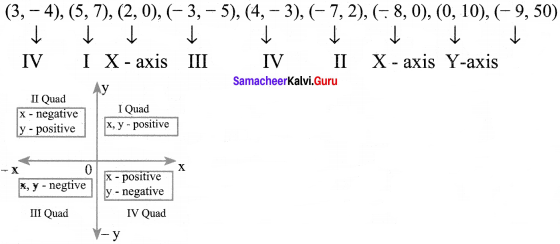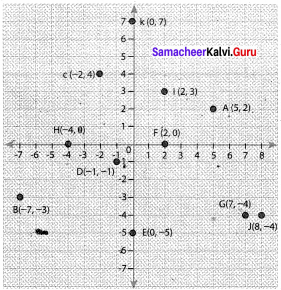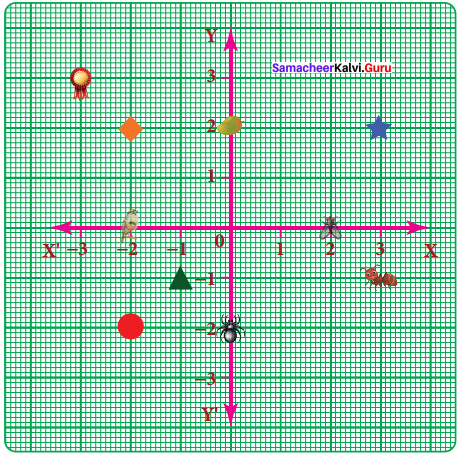# Samacheer Kalvi 8th Maths Solutions Term 2 Chapter 2 Algebra Ex 2.3

Students can Download Maths Chapter 2 Algebra Ex 2.3 Questions and Answers, Notes Pdf, Samacheer Kalvi 8th Maths Book Solutions Guide Pdf helps you to revise the complete Tamilnadu State Board New Syllabus and score more marks in your examinations.

## Tamilnadu Samacheer Kalvi 8th Maths Solutions Term 2 Chapter 2 Algebra Ex 2.3

Question 1.
Fill in the blanks:

Question (i)
X – axis and Y – axis intersect at ……..
Origin (0, 0)Question (ii)
The coordinates of the point in third quadrant are always ……….
negatives

Question (iii)
(0, -5) point lies on ………. axis.
y – axis.

Question (iv)
The x coordinate is always ……… on the y – axis.
Zero

Question (v)
Coordinates are the same for a line parallel to Y – axis.
X.

Question 2.
Say True or False:

Question (i)
(-10, 20) lies in the second quadrant.
True
Hint:
(-10, 20)
x = – 10,
y = 20
∴ (- 10, 20) lies in second quadrant – TrueQuestion (ii)
(-9, 0) lies on the x – axis.
True
Hint:
(-9, 0) on x – axis, Y – coordinate is always zero.
∴ (-9, 0) lies on x – axis – True

Question (iii)
The coordinates of the origin are (1, 1).
False
Hint:
Coordinate of origin is (0, 0), not (1, 1). Hence – False

Question 3.
Find the quadrants without plotting the points on a graph sheet.
(3, -4), (5, 7), (2, 0), (- 3, – 5), (4, – 3), (- 7, 2), (- 8, 0), (0,10), (- 9, 50).
Solution:• If x & y coordinate are positive – I quad
• If x is positive, y is negative – IV quad
• If x is negative, y is positive – II quad
• If both are negative, then – III quad

Question 4.
Plot the following points in a graph sheet.
A(5, 2), B(- 7, – 3), C(- 2, 4), D(- 1, – 1), E(0, – 5), F(2, 0), G(7, – 4), H(- 4, 0), 1(2,3), J(8, – 4) K (0, 7).
Solution:Question 5.
Use the grid graph to determine the coordinates where each figure is located.a) Star ……..
b) Bird ……..
c) Red Circle ……..
d) Diamond ……..
e) Triangle ……..
f) Ant ……..
g) Mango ……..
h) Housefly ……..
i) Medal ……..
j) Spider ……..
Solution:
a) Star (3, 2)
b) bird (-2, 0)
c) Red Circle (-2, -2)
d) Diamond (-2, 2)
e) Triangle (-1, -1)
f) Ant (3, -1)
g) Mango (0, 2)
h) Housefly (2, 0)
i) Medal (-3, 3)
j) Spider (0, -2)